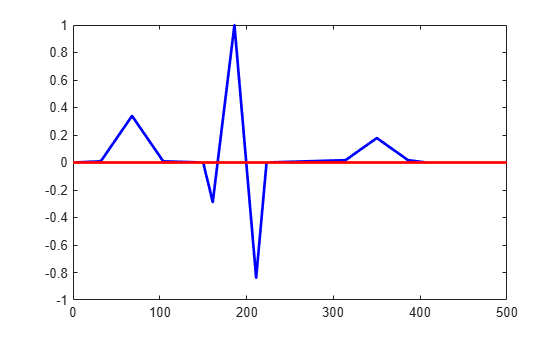# dsp.ZeroCrossingDetector

Detect zero crossings

## Description

The `dsp.ZeroCrossingDetector` System object™ counts the number of times the signal crosses zero, or changes sign. To detect if a signal in a given channel crosses zero, the object looks for the following conditions, where, xi-l is the current signal value and xi-1 is the previous signal value:

• xi < 0 and xi-1 > 0

• xi > 0 and xi-1 < 0

• For some positive integer L, xi < 0, xi-l = 0, and xi-L-1 > 0, where $0\le l\le L$.

• For some positive integer L, xi > 0, xi-l = 0, and xi-L-1 < 0, where $0\le l\le L$.

For the first input value, xi-1 and xi-2 are zero.

To count the number of times a signal crosses zero or changes sign:

1. Create the dsp.ZeroCrossingDetector object.

2. Call the object with arguments, as if it were a function.

## Creation

### Syntax

``zcd = dsp.ZeroCrossingDetector``

### Description

example

````zcd = dsp.ZeroCrossingDetector` returns a zero crossing detection object that calculates the number of times the signal crosses zero.```

## Usage

### Syntax

``zcdOut = zcd(input)``

### Description

example

````zcdOut = zcd(input)` calculates the number of zero crossings of the input. Each column of the input is treated as an independent channel.```

### Input Arguments

expand all

Data input whose zero crossings are counted by the object, specified as a vector or a matrix.

Example: `rand(20,1)-0.3`

Example: `rand(20,2)-0.3`

Data Types: `single` | `double` | `int8` | `int16` | `int32` | `int64` | `uint8` | `uint16` | `uint32` | `uint64` | `fi`

### Output Arguments

expand all

Number of zero crossings in the data input, returned as one of the following:

• scalar –– When the data input is a column vector, the scalar output is the number of zero crossings in the data input..

• row vector –– When the data input is a matrix, each element in the row vector output is the number of zero crossings in the corresponding column of the data input.

Example: `10`

Example: [`9,6`]

Data Types: `uint32`

## Object Functions

To use an object function, specify the System object as the first input argument. For example, to release system resources of a System object named `obj`, use this syntax:

`release(obj)`

expand all

 `step` Run System object algorithm `release` Release resources and allow changes to System object property values and input characteristics `reset` Reset internal states of System object

## Examples

collapse all

Note: If you are using R2016a or an earlier release, replace each call to the object with the equivalent `step` syntax. For example, `obj(x)` becomes `step(obj,x)`.

Find the number of zero crossings in electrocardiogram data.

```EcgData = ecg(500)'; zcd = dsp.ZeroCrossingDetector; numZeroCross = zcd(EcgData)```
```numZeroCross = uint32 4 ```
`plot(1:500,EcgData,'b',[0 500],[0 0],'r','linewidth',2)`## Version History

Introduced in R2012a